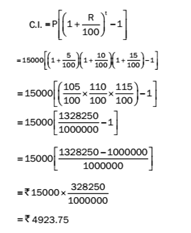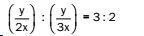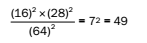# RRB ALP 2018 Practice Test Papers | Arithmetic Questions (Day-20)

Dear Aspirants, Here we have given the Important RRB ALP & Technicians Exam 2018 Practice Test Papers. Candidates those who are preparing for RRB ALP 2018 can practice these Arithmetic Questions to get more confidence to Crack RRB 2018 Examination.

[WpProQuiz 1755]

### Click “Start Quiz” to attend these Questions and view Explanation

1. A sum of money becomes 5 times of itself in 16 years. What is the rate of interest?
1. 25%
2. 18%
3. 16%
4. 17%
1. Calculate the compound interest on Rs.15000 in 3 years, when the rates of interest for successive years are 5%, 10% and 15%.
1. Rs.2520
2. Rs.4923.75
3. Rs.4258.50
4. Rs.4653.80
1. The ratio of speeds of two cars is 2: 3. What will be the ratio of the time required to travel the same distance?
1. 5: 3
2. 2: 3
3. 3: 4
4. 3: 2
1. The HCF of two numbers in the ratio 2: 7 is 23. Find their LCM.
1. 322
2. 46
3. 151
4. 197
1. The product of two numbers is 16464. If their LCM is 1176, then find their HCF.
1. 84
2. 14
3. 28
4. 56
1. Jay and Vijay start cycling from the same point in opposite directions at the speed of 20 kmph and 24 kmph, respectively. What will be the distance between them after 15 minutes?
1. 1 km
2. 11 km
3. 5 km
4. 13 km
1. An amount of money is to be divided between A, B and C in the ratio 4: 7: 11. If the difference between the shares of B and C is Rs.9600, what will be the difference between the shares of A and B?
1. Rs.8000
2. Rs.7200
3. Rs.7500
4. Rs.6800
1. Reema started a business of bread manufacturing with Rs.4500. After 4 months, Anshu joined Reema as her business partner. After 1 year, the profit was divided in the ratio 5: 6. How much did Anshu contribute?
1. Rs.2000
2. Rs.5236
3. Rs.4125
4. Rs.8100
1. In what ratio should water be mixed with milk costing Rs.15 per litre to obtain a mixture worth Rs.9 per litre?
1. 1: 2
2. 4: 3
3. 2: 3
4. 5: 7
1. The value of (16)2x (28)2/ (64)2 is
1. 59
2. 56
3. 78
4. 49

Let principal = Rs.x

∴ Amount (A) = 5x

S.I. = A – P

S.I. = 5x – x = Rs.4x

4x =x*16*r/100

Rate% = 25%Let the speeds of two cars be 2x km/h and 3x km/h and the distance be y km. ∴ Time required = y/2x h and y/3xh ∴ The ratio of the time required =Since the numbers are in the ratio 2: 7 and HCF is 23, the required LCM = 2 × 7 × 23 = 322

Product of HCF and LCM of two numbers = Product of the two numbers

HCF = Product of two numbers ÷ LCM = 16464 ÷ 1176 = 14

Distance travelled by Jay in 15 min = 20 × 15 60 km = 5 km

Distance travelled by Vijay in 15 min = 24 × 15 /60 km = 6 km

∴ Total distance between them = 5 km + 6 km = 11 km

Let the shares of A, B and C be 4x, 7x and 11x, respectively.

According to the question, 11x – 7x = 9600

4x = 9600

x = 2400 Difference in the share of A and B = 3x = 3 × 2400 = Rs.7200

Suppose Anshu invested Rs.x in a partnership business. According to the question,

Investment of Reema: Investment of Anshu = Reema’s share of profit: Anshu’s share of profit

(4500 × 12): (x × 8) = 5: 6

x = Rs.8100

C.P. of 1 litre of water = 0

C.P. of 1 litre of milk = Rs.15

Price of 1 litre mixture = Rs. 9

Required ratio = (15 – 9): (9 – 0) = 6: 9 = 2: 3RRB ALP 2018 Practice Test Papers | Arithmetic Questions (Day-1)

RRB ALP 2018 Practice Test Papers | Arithmetic Questions (Day-2)

RRB ALP 2018 Practice Test Papers | Arithmetic Questions (Day-3)

RRB ALP 2018 Practice Test Papers | Arithmetic Questions (Day-4)

RRB ALP 2018 Practice Test Papers | Arithmetic Questions (Day-5)

RRB ALP 2018 Practice Test Papers | Arithmetic Questions (Day-6)

RRB ALP 2018 Practice Test Papers | Arithmetic Questions (Day-7)

RRB ALP 2018 Practice Test Papers | Arithmetic Questions (Day-8)

RRB ALP 2018 Practice Test Papers | Arithmetic Questions (Day-9)

RRB ALP 2018 Practice Test Papers | Arithmetic Questions (Day-10)

RRB ALP 2018 Practice Test Papers | Arithmetic Questions (Day-11)

RRB ALP 2018 Practice Test Papers | Arithmetic Questions (Day-12)

RRB ALP 2018 Practice Test Papers | Arithmetic Questions (Day-13)

RRB ALP 2018 Practice Test Papers | Arithmetic Questions (Day-14)

RRB ALP 2018 Practice Test Papers | Arithmetic Questions (Day-15)

RRB ALP 2018 Practice Test Papers | Arithmetic Questions (Day-16)

RRB ALP 2018 Practice Test Papers | Arithmetic Questions (Day-17)

RRB ALP 2018 Practice Test Papers | Arithmetic Questions (Day-18)

RRB ALP 2018 Practice Test Papers | Arithmetic Questions (Day-19)